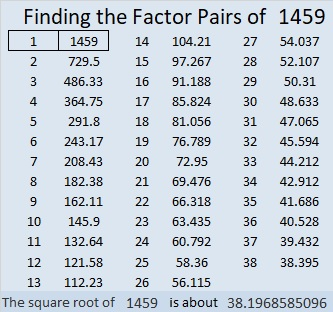# 1459 and Level 4

Contents

### Today’s Puzzle:

Can you place the numbers 1 to 10 in both the first column and the top row so that those numbers are the factors of the given clues? You might surprise yourself with how well you do!### Factors of 1459:

• 1459 is a prime number.
• Prime factorization: 1459 is prime.
• 1459 has no exponents greater than 1 in its prime factorization, so √1459 cannot be simplified.
• The exponent in the prime factorization is 1. Adding one to that exponent we get (1 + 1) = 2. Therefore 1459 has exactly 2 factors.
• The factors of 1459 are outlined with their factor pair partners in the graphic below.How do we know that 1459 is a prime number? If 1459 were not a prime number, then it would be divisible by at least one prime number less than or equal to √1459. Since 1459 cannot be divided evenly by 2, 3, 5, 7, 11, 13, 17, 19, 23, 29, 31, or 37, we know that 1459 is a prime number.

### Other Facts about the number 1459:

1459 is the difference of two squares:
730² – 729² = 1459

From OEIS.org we learn this chilling cubic fact:
1³ + 4³ + 5³ + 9³ = 919, and
9³ + 1³ + 9³ = 1459

This site uses Akismet to reduce spam. Learn how your comment data is processed.